Test: Plane Table Surveying & Calculation of Area & Volume - 2

# Test: Plane Table Surveying & Calculation of Area & Volume - 2

Test Description

## 10 Questions MCQ Test GATE Civil Engineering (CE) 2023 Mock Test Series | Test: Plane Table Surveying & Calculation of Area & Volume - 2

Test: Plane Table Surveying & Calculation of Area & Volume - 2 for Civil Engineering (CE) 2022 is part of GATE Civil Engineering (CE) 2023 Mock Test Series preparation. The Test: Plane Table Surveying & Calculation of Area & Volume - 2 questions and answers have been prepared according to the Civil Engineering (CE) exam syllabus.The Test: Plane Table Surveying & Calculation of Area & Volume - 2 MCQs are made for Civil Engineering (CE) 2022 Exam. Find important definitions, questions, notes, meanings, examples, exercises, MCQs and online tests for Test: Plane Table Surveying & Calculation of Area & Volume - 2 below.
Solutions of Test: Plane Table Surveying & Calculation of Area & Volume - 2 questions in English are available as part of our GATE Civil Engineering (CE) 2023 Mock Test Series for Civil Engineering (CE) & Test: Plane Table Surveying & Calculation of Area & Volume - 2 solutions in Hindi for GATE Civil Engineering (CE) 2023 Mock Test Series course. Download more important topics, notes, lectures and mock test series for Civil Engineering (CE) Exam by signing up for free. Attempt Test: Plane Table Surveying & Calculation of Area & Volume - 2 | 10 questions in 30 minutes | Mock test for Civil Engineering (CE) preparation | Free important questions MCQ to study GATE Civil Engineering (CE) 2023 Mock Test Series for Civil Engineering (CE) Exam | Download free PDF with solutions
 1 Crore+ students have signed up on EduRev. Have you?
Test: Plane Table Surveying & Calculation of Area & Volume - 2 - Question 1

### The resected position of plane-table station from three known position is unreliable if the station lies

Test: Plane Table Surveying & Calculation of Area & Volume - 2 - Question 2

### Which of the following methods of plane tabling also known as “graphic triangulation”

Test: Plane Table Surveying & Calculation of Area & Volume - 2 - Question 3

### The quick and most accurate method to solve three point problem in resection type of plane tabling is

Test: Plane Table Surveying & Calculation of Area & Volume - 2 - Question 4

The ‘fix’ of a plane table with three known points is good if the instrument station lies

Test: Plane Table Surveying & Calculation of Area & Volume - 2 - Question 5

Consider the following steps
1. Using Lehmann's rule, an improved position of station point is obtained
2. Procedure is repeated till the triangle of error is reduced to a point
3. Triangle of error is obtained
4. The plane table is levelled over the station point
5. Three resection tines are drawn from three well defined station points

The correct sequence of "Lehman’s Procedure" for solving the three point problem is

Test: Plane Table Surveying & Calculation of Area & Volume - 2 - Question 6

Consider the following statements regarding Plane Table surveying
1. It is less accurate than chain surveying
2. It is not necessary to do accurate centering of plane table for small scale surveys
3. Compass rule may be made use of for adjusting the plane table traverse
4. From the instrument station, resectors are drawn to plot the position of objects in the field

Which of these statements is/are correct?

Test: Plane Table Surveying & Calculation of Area & Volume - 2 - Question 7

Consider the following statements pertaining to plane table survey
1. Two-point problem is solved by mechanical method.
2. Three-point problem is solved by Bessel’s method
3. In two-point problem, auxiliary station is required

Which of these statements is/are correct?

Test: Plane Table Surveying & Calculation of Area & Volume - 2 - Question 8

Which one of the following instruments is used in plane table surveying for the measurement of horizontal and vertical distances directly?

Test: Plane Table Surveying & Calculation of Area & Volume - 2 - Question 9

In the given formula formats, L is the length of a base line split into ‘n’ equal segments each of length ‘d’. O1, O2 .......On+1 are the ordinates at the sequential ends of the segments and M1, M2....Mn are the mid-ordinates of successive segments. Which of the following pairs of rules and the formulae for computation of the area standing on the base line are correctly matched?
1. Mid - ordinate Rule ...............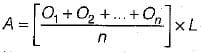2. Average - ordinate Rule ......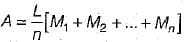3. Trapezoidal Rule........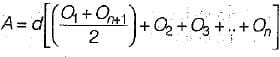4. Simpsons’ rule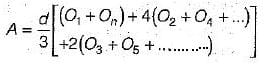Select the correct answer using the codes given below

Detailed Solution for Test: Plane Table Surveying & Calculation of Area & Volume - 2 - Question 9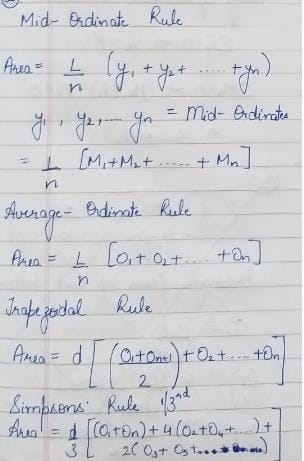Test: Plane Table Surveying & Calculation of Area & Volume - 2 - Question 10

Which one of the following methods estimates best the area of an irregular and curved boundary?

Detailed Solution for Test: Plane Table Surveying & Calculation of Area & Volume - 2 - Question 10

Mid-ordinate and Average ordinate methods are used with the assumption that the boundaries between the extremities of the ordinates are straight lines.
Trapezoidal method is based on the assumption that the figures are trapezoids. The method is more accurate than the above two methods.
Simpson’s method assumes that the short lengths of the boundaries between the ordinates are parabolic arcs. This method is more useful when the boundary line departs considerably from the straight line. The results obtained by use of Simpson’s method in all cases are more accurate.

## GATE Civil Engineering (CE) 2023 Mock Test Series

25 docs|282 tests
 Use Code STAYHOME200 and get INR 200 additional OFF Use Coupon Code
Information about Test: Plane Table Surveying & Calculation of Area & Volume - 2 Page
In this test you can find the Exam questions for Test: Plane Table Surveying & Calculation of Area & Volume - 2 solved & explained in the simplest way possible. Besides giving Questions and answers for Test: Plane Table Surveying & Calculation of Area & Volume - 2, EduRev gives you an ample number of Online tests for practice

## GATE Civil Engineering (CE) 2023 Mock Test Series

25 docs|282 tests